﻿ Expressions and Formulas
CalcTime - Easy as ABC 123 ...

Expressions and Formulas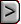Expressions and Formulas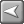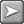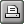Expressions and Formulas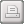Expressions

An expression is made up of two types of components: operands and operators. Operators perform an operation (such as addition, subtraction, etc.) on one or more operands of the expression. Operands are the components on which operations are performed. Operands either contain or return a value. Constants, data dictionary columns, memory variables, and functions are examples of operands. An operand can be made up of more than one component, such as a function and its parameters.

The Frame Editor lets you choose operators and operands, and then insert them into the Statement line.

The sections below lists all the components used in Clarion expressions.

Math Operators

+        Plus sign: Adds two operands together.

-        Minus sign: Subtracts one operand from another.

*        Asterisk: Multiplies one operand by another.

/        Slash: Divides one operand by another.

%        Percent sign: Returns the remainder from a division operation (modulus division).

^        Caret: Raises one operand to the power of the other.

( )        Parentheses: Groups components together within an expression.

Logical (Boolean) Operators

=        Equal: Evaluates whether one expression is equal to the other.

<        Less Than: Evaluates whether one expression is less than the other.

>        Greater Than: Evaluates whether one expression is greater than the other.

<>        Not Equal: Evaluates whether one expression is not equal to the other.

>=        Greater or Equal: Evaluates whether one expression is greater than or equal to the other.

<=        Less or Equal: Evaluates whether one expression is less than or equal to the other.

AND        Connects two logical expressions together. For an expression containing an AND to be true, both expressions of the AND must be true.

OR        Connects two logical expressions together. An expression containing an OR is true if either expression of the OR is true.

XOR        Connects two logical expressions together. An XOR expression is true if either expression is true, but not both.

NOT        Reverses the evaluation of an expression.

~        Reverses the evaluation of an expression.

String Operators

&        Ampersand: Appends one text string to another.

Operands

Data ~Includes data dictionary columns, global, and local memory variables.

Functions ~All of the built-in functions of the Clarion language. These functions all perform some operation on parameters (other operands) and return a value.

User ~Any FUNCTION in your application. These functions perform some operation on parameters (other operands) and return a value.

Constant Text ~You can type constant text surrounded in single quotes ( ‘A’ ) on the Statement line.

Constant Number ~You can type constant numbers on the Statement line. Constant numbers can be represented in any valid format, such as Decimal (1 or 1.2345), Scientific Notation ( 22e4), Binary (0101b), or Hexadecimal (1AFFh).

Example Expressions

Lastname & ‘, ‘ & FirstName                !concatenated string constants & variables

ABS(Amount)        * 100                        !function, numeric variable & constant

TaxCode = True AND Amount > .25        !Boolean with variables & constants